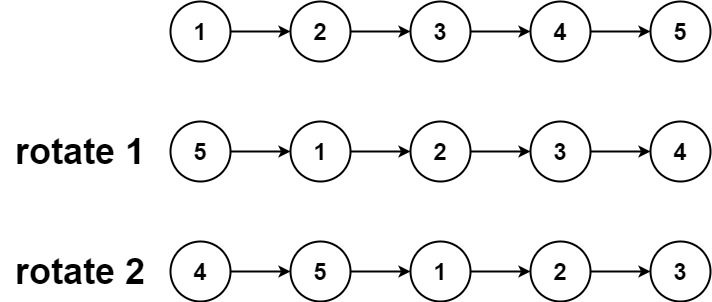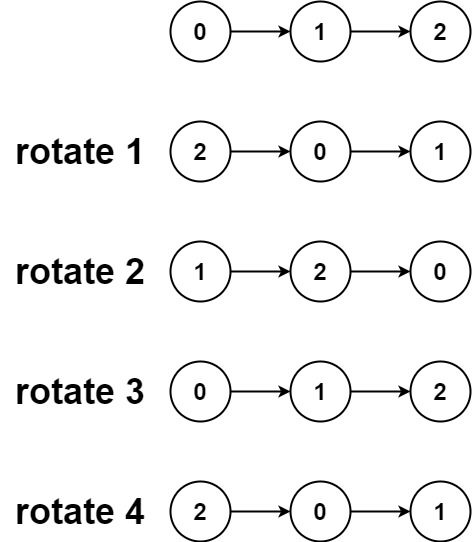# Question

Formatted question description: https://leetcode.ca/all/61.html

Given the head of a linked list, rotate the list to the right by k places.

Example 1:Input: head = [1,2,3,4,5], k = 2
Output: [4,5,1,2,3]


Example 2:Input: head = [0,1,2], k = 4
Output: [2,0,1]


Constraints:

• The number of nodes in the list is in the range [0, 500].
• -100 <= Node.val <= 100
• 0 <= k <= 2 * 109

# Algorithm

First traverse the original linked list to get the length n of the linked list, and then take the remainder of k to n, so that k must be less than n.

Use the fast and slow pointers to solve, the fast pointer first walks k steps, and then the two pointers go together, when the fast pointer reaches the end , The next position of the slow pointer is the head node of the new order, so that the linked list can be rotated.

# Code

• 
public class Rotate_List {

/**
* public class ListNode {
*     int val;
*     ListNode next;
*     ListNode(int x) { val = x; }
* }
*/
public class Solution {
public ListNode rotateRight(ListNode head, int k) {

}

// k could be larger than list-length
int length = 0;
while (p != null) {
length++;
p = p.next;
}

// avoid circle rotate
k = k % length;

if (k == 0) {
}

// two pointers, j is k behind i
while (k > 0) {
i = i.next;
k--;
}

while (i.next != null) { // i will stop at last node, j will stop at after-rotate end node

i = i.next;
j = j.next;
}

ListNode newHead = j.next; // @note: here, hidden assumption is "j.next != null” . eg: input: list=, k=0
j.next = null; // cut

}

}

public class Solution_make_circle {

public ListNode rotateRight(ListNode head, int n) {

if (head == null || head.next == null || n == 0)

// compare list length with k, what if k is extremely large
int count = 1;
while (ptail.next != null) {
count++;
ptail = ptail.next;
}

int cutoff = count - (n % count);

ptail.next = head; // @note: connect head tail, making it circular list

for (int i = 0; i < cutoff; i++) {
ptail = ptail.next;
}

ptail.next = null;
}
}

}

############

/**
* public class ListNode {
*     int val;
*     ListNode next;
*     ListNode() {}
*     ListNode(int val) { this.val = val; }
*     ListNode(int val, ListNode next) { this.val = val; this.next = next; }
* }
*/
class Solution {
public ListNode rotateRight(ListNode head, int k) {
if (k == 0 || head == null || head.next == null) {
}
int n = 0;
for (ListNode cur = head; cur != null; cur = cur.next) {
++n;
}
k %= n;
if (k == 0) {
}
while (k-- > 0) {
fast = fast.next;
}
while (fast.next != null) {
slow = slow.next;
fast = fast.next;
}

ListNode start = slow.next;
slow.next = null;
return start;
}
}

• // OJ: https://leetcode.com/problems/rotate-list/
// Time: O(N)
// Space: O(1)
class Solution {
int ans = 0;
return ans;
}
public:
ListNode* rotateRight(ListNode* head, int k) {
k %= len;
if (k == 0) return head;
while (k--) q = q->next;
while (q->next) p = p->next, q = q->next;
auto ans = p->next;
p->next = nullptr;
return ans;
}
};

• # Definition for singly-linked list.
# class ListNode:
#     def __init__(self, val=0, next=None):
#         self.val = val
#         self.next = next
class Solution:
def rotateRight(self, head: ListNode, k: int) -> ListNode:
if k == 0 or head is None or head.next is None:
while cur:
n, cur = n + 1, cur.next
k %= n
if k == 0:

for _ in range(k):
fast = fast.next
while fast.next:
slow, fast = slow.next, fast.next

start = slow.next
slow.next = None
return start

############

# class ListNode(object):
#     def __init__(self, x):
#         self.val = x
#         self.next = None

class Solution(object):
"""
:type k: int
:rtype: ListNode
"""
l = 1
while p.next:
l += 1
p = p.next
k = k % l
if k == 0:
k = l - k % l - 1
print
k
while k > 0:
pp = pp.next
k -= 1
pp.next = None


• /**
* public class ListNode {
*     public int val;
*     public ListNode next;
*     public ListNode(int val=0, ListNode next=null) {
*         this.val = val;
*         this.next = next;
*     }
* }
*/
public class Solution {
public ListNode RotateRight(ListNode head, int k) {
if (k == 0 || head == null || head.next == null)
{
}
var n = 0;
for (ListNode cur = head; cur != null; cur = cur.next)
{
++n;
}
k %= n;
if (k == 0)
{
}
while (k-- > 0)
{
fast = fast.next;
}
while (fast.next != null)
{
slow = slow.next;
fast = fast.next;
}

ListNode start = slow.next;
slow.next = null;
return start;
}
}

• /**
* type ListNode struct {
*     Val int
*     Next *ListNode
* }
*/
func rotateRight(head *ListNode, k int) *ListNode {
}
n := 0
for cur != nil {
cur = cur.Next
n++
}
k %= n
if k == 0 {
}
for i := 0; i < k; i++ {
fast = fast.Next
}
for fast.Next != nil {
fast = fast.Next
slow = slow.Next
}
ans := slow.Next
slow.Next = nil